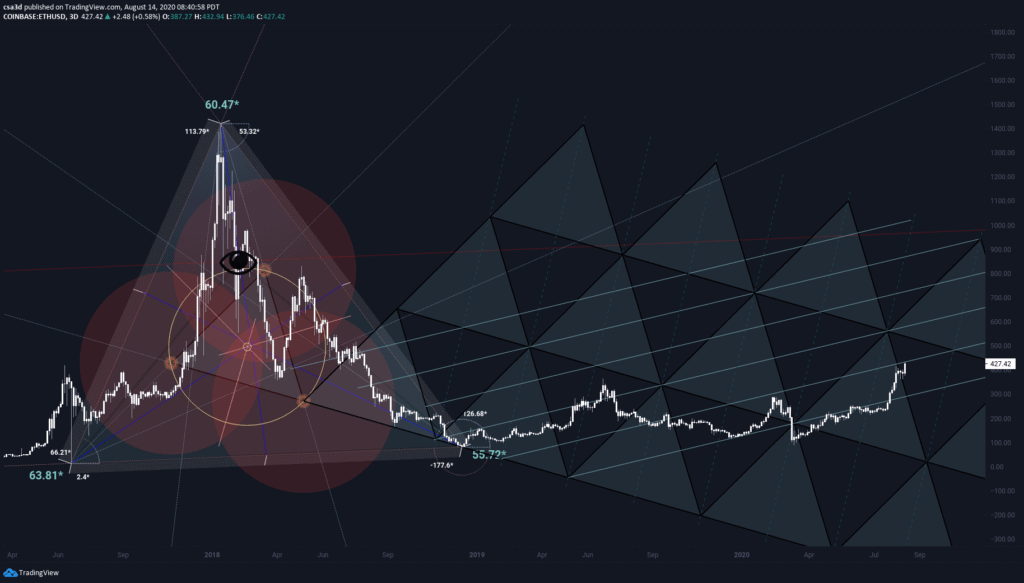# Triangle Tutorial with CSA3D

The chart below is the basis for the tutorial today.

This tutorial has been made available thanks to CSA3D.

Let’s dive in…

We will see that if we divide each interior angle of any triangle into angular thirds, the intersections become the hidden triangle vertex.

Squaring the large triangle… however, nothing is aligned perfectly for the top to fit within my circle, but I know however that the technique is still valid.

Split each side into thirds and you will find a hidden equilateral triangle.